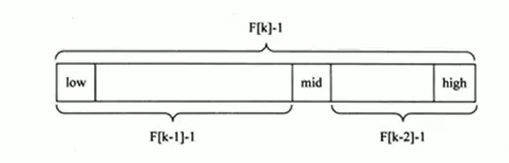# 查找算法——斐波那契查找法（黄金分割法）

## 查找算法——斐波那契查找法（黄金分割法）

1. 基本介绍：斐波那契中除去第一项的相邻的两项比值近似为黄金分割点的比值。在二分法的基础上，中点的确定采用黄金分割点的定义来求得。
• 黄金分割点的定义：其中一部分的比值与全程的比值等于另一部分与这部分的比值，即：a / (a + b) = b/ a。
1. 原理讲解：
• 中间点采用公式 mid = left + F(k - 1) -1;
left mid right
left到（mid - 1）这段为F(k - 1) - 1 left到（mid - 1）这段为F(k - 1) - 1 由（mid + 1）到right这段为 F(k - 2) - 1 由（mid + 1）到right这段为 F(k - 2) - 1• 对于F(k - 1) - 1的理解：
• 斐波那契公式：F（K） = F（K- 1）+ F（K- 2）>> 两边同时减一》》F（K）- 1 = F（K- 1）- 1+ F（K- 2）- 1 + 1》》F（K）- 1 = F（K- 1）- 1+ F（K- 2）- 1 + mid
• 但是有序数组的长度可能不一定恰好等于F（K）- 1 ，所以需要将原来的顺序表长度n增加到F（K）- 1，可调用黄金分割点。该处的K值只要使得F（K）- 1大于或者是等于有序数组长度就可以，然后有序数组在扩容至对应的长度。
1. 思想分析
• 生成对应的斐波那契数列，用于查找赋值
• 获取到斐波那契分割数值的下标，当原数组的长度小于斐波那契数，那就扩容，用最后一位数字进行填充
• 然后使用while根据设置的mid点进行比较
• 比mid小，那就是中间值的前半部分；比起大就是后面部分
1. 代码实现：
 package faboccisearch;

import java.util.Arrays;

public class FabocciSearch {
public static int max = 20;
public static void main(String[] args) {
int[] arr = {1,2,4,6,8,56};
System.out.println(fabocciSearch(arr,56));
}
//因为是采用斐波那契数列中的值,所以先获取数列

//返回斐波那契数列,用于数组查找
public static int[] fabocci(){
int[] arr = new int[max];
arr = 1;
arr = 1;
for(int i = 2;i < arr.length;i ++){
arr[i] = arr[i -1] + arr[i - 2];
}
return arr;
}
public static int fabocciSearch(int[] arr,int searchVal){
int left = 0;
int right = arr.length - 1;
int[] f = fabocci();
int k = 0;
int mid = 0;
//找到对应的比原始数组长度大的fabocci数组
while ((f[k]) < arr.length){
k ++;
}
//根据所给的fabocci数将原始数组扩容
int[] temp= Arrays.copyOf(arr,f[k]);
//为什么是扩容成f(k)?
//因为是将整个数组长度变成斐波那契数列,f(k) = f(k - 1) + f(k - 2);
//>>f(k) - 1 = f(k -1) - 1 + f(k - 2) - 1 + (mid)1
//根本上还是扩容成f(k)
//将扩容的部分全部变成最大值
for (int i = arr.length;i < temp.length;i ++){
temp[i] = arr[arr.length - 1];
}
//生成对应的重点进行比较
while (left <= right){
mid = left + f[k - 1] - 1;
//f(k) - 1 = f(k -1) - 1 + f(k - 2) - 1 + (mid)1
//由mid1区分f(k -1) - 1 和f(k - 2) - 1 两者
if (searchVal < temp[mid]){
right = mid - 1;
k --;
}else if(searchVal > temp[mid]){
left = mid + 1;
k -= 2;
}else{
//因为在比较的时候,用的是temp而不是arr.
//虽然二者的索引值是相同的,但是他们的长度不一样,最大值的做因可能是很多种情况
if (mid < arr.length - 1){
return mid;
}else{
return arr.length - 1;
}
}
}
return -1;
}
}

1. 分析与总结
• 谁能告诉我，斐波那契查找法是更快？还是更省空间？似乎都不是，仅仅是显示出计算机人的浪漫而已！但是于我而言，还是一种逻辑思维的锻炼，实际作用不是很大！还不如插入查找。
• 问：为什么是否执行的判定条件会加上等号？
• 答：二分法查找，mid = （left + right）/ 2，由于整除的特性，他是默认偏左的，对于末尾置的值是查找不到的，所以加等号。插入法查找，mid = left + (key - arr) /(arr[arr.length -1] - arr)*arr.length,会根据自身的值决定自身的到起点的距离，不存在偏左或者偏右的情况，一步到位；斐波那契法查找，由于斐波那契数列的特性，往左会导致值偏右，往右会导致值偏左，所以分别检测不到最大值和最小值，所以必须加等号。

©️2019 CSDN 皮肤主题: 游动-白 设计师: 上身试试